# Congruence of Triangles Class 7 Notes: Chapter 7

According to the CBSE Syllabus 2023-24, this chapter has been removed from NCERT Class 7 Maths textbook.

## Introduction to Congruent Triangles

### Congruent Figures

• Congruent figures are exactly equal in size and shape.

To know more about Congruent Figures, visit here.

### Congruent Triangles

• If all the sides and angles of a triangle are equal to the corresponding sides and angles of another triangle, then both triangles are said to be congruent.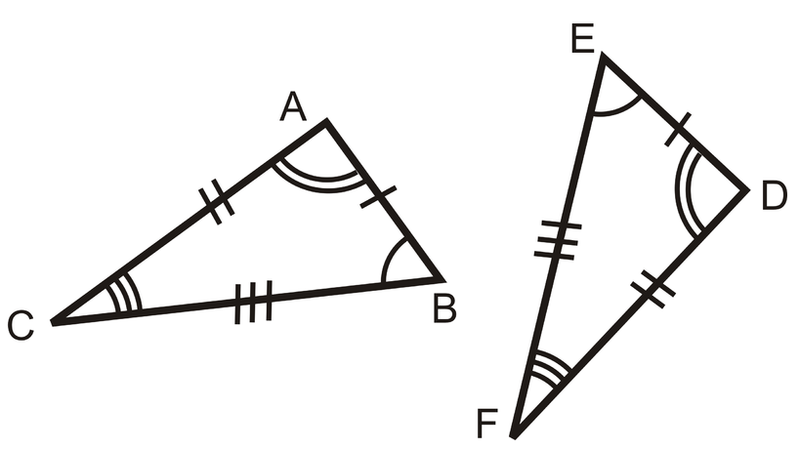Here, ABCDEF

To know more about the Congruence of Triangle, visit here.

#### For more information on The Introduction to Congruent Triangles, watch the below video## Criteria for Congruency

### SSS Criteria for Congruency

• If, under a given correspondence, the three sides of one triangle are equal to the three corresponding sides of another triangle, then the triangles are congruent.
​​​​​​​​​​​​​​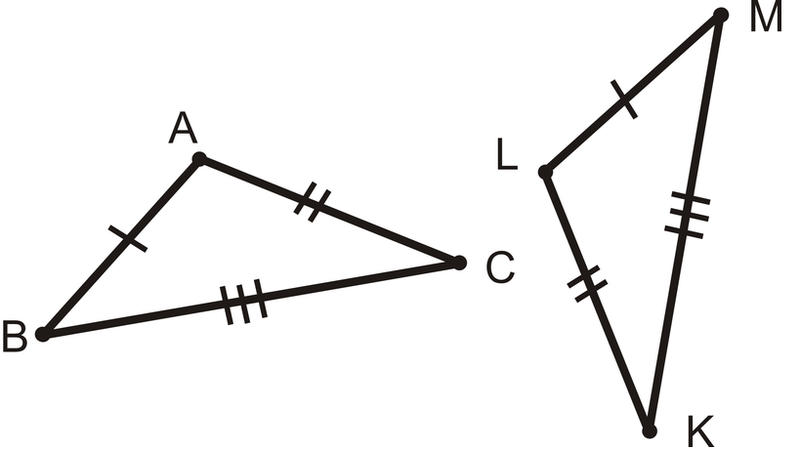### SAS Criteria for Congruency

• If, under a correspondence, two sides and the angle included between them of a triangle are equal to two corresponding sides and the angle included between them of another triangle, then the triangles are congruent.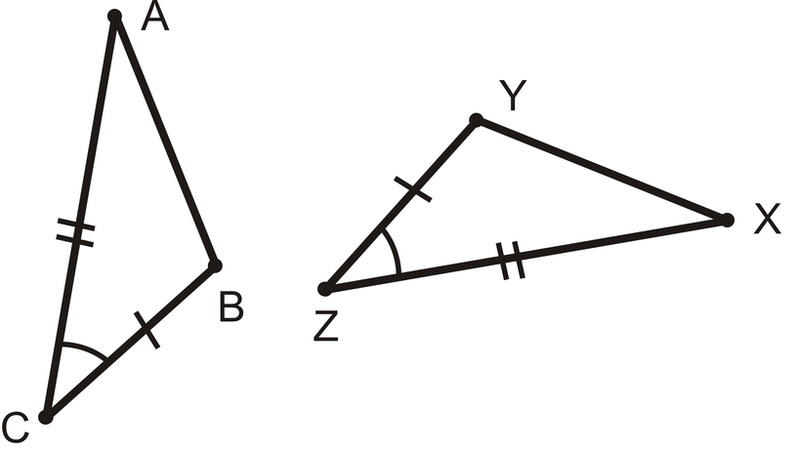### ASA Criteria for Congruency

• If, under a correspondence, two angles and the included side of a triangle are equal to two corresponding angles and the included side of another triangle, then the triangles are congruent.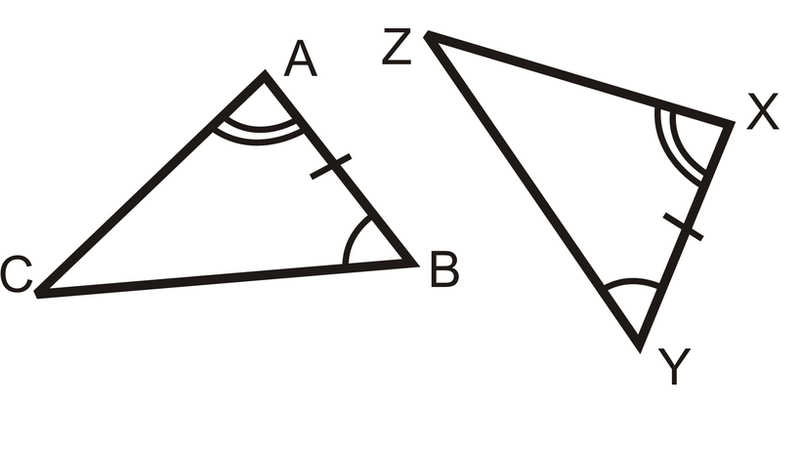### AAS Criteria for Congruency

AAS Rule: Triangles are congruent if two pairs of corresponding angles and a pair of opposite sides are equal in both triangles.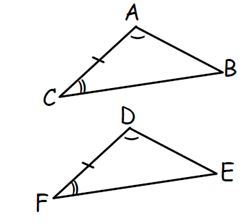### Why are SSA and AAA congruency rules not valid?

• Two triangles with equal corresponding angles need not be congruent. In such a correspondence, one of them can be an enlarged copy of the other. Therefore AAA congruency is not valid.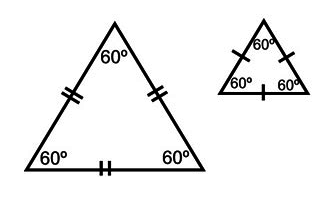•  If two triangles have two congruent sides and a congruent non-included angle, then triangles are not necessarily congruent. Therefore, SSA congruency is not valid.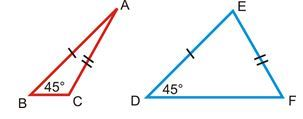### RHS Criteria for Congruency

• If, under a correspondence, the hypotenuse and one side of a right-angled triangle are respectively equal to the hypotenuse and one side of another right-angled triangle, then the triangles are congruent.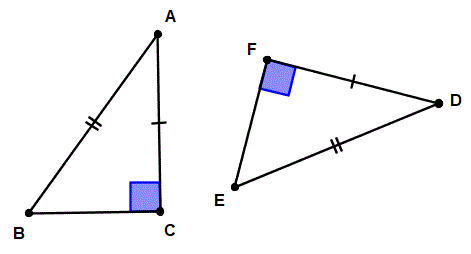To know more about RHS and SSS Congruency, visit here.

### Criteria for Congruency

Criteria for the Congruency of two triangles are:
(i) SSS Rule
(ii) SAS Rule
(iii) ASA Rule
(iv) RHS Rule

## Frequently Asked Questions on CBSE Class 7 Maths Notes Chapter 7 Congruence of Triangles

Q1

### What are some of the facts of triangles?

A triangle has 1. three sides, three vertices and three angles 2. The sum of the three interior angles of a triangle is always 180° 3. The sum of the length of two sides of a triangle is always greater than the length of the third side.

Q2

### What is the angle sum property?

The angle sum property of a triangle says that the sum of its interior angles is equal to 180°.

Q3

### What are some of the uses of triangles?

Triangles can be used to make trusses. Trusses are used in many structures, such as roofs, bridges and buildings.